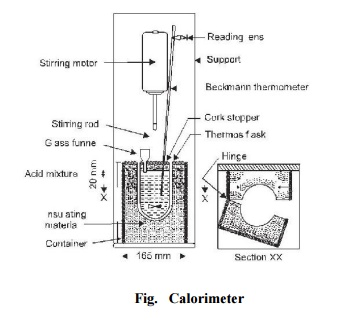Home | | Construction Materials | Heat of hydration

# Heat of hydrationHeat is evolved during hydration of cement, the amount being dependent on the relative quantities of the clinker compounds.

Heat of hydration

Heat is evolved during hydration of cement, the amount being dependent on the relative quantities of the clinker compounds.

Importance: The evolution of heat causes an increase in temperature of the concrete, being greatest in mass concreting. Since the cooling of a mass of concrete can only occur from surfaces exposed to atmosphere the temperature of the interior is higher than that at the surface and also there is a rapid increase in strength in the interior than at the surface. Shrinkage cracks may result from stresses, induced by cooling of the surface while the interior of concrete is still at higher temperature. However, in practice, the heat evolution may be taken to its advantage in cold weather provided the concrete is warm at the time of placing and excessive heat loss is prevented by suitable lagging.

Test Procedure: The apparatus used to determine the heat of hydration of cement is known as calorimeter and is shown in Fig. 32. 60 g of cement and 24 ml of distilled water are mixed for 4 minutes at temperature 15 o -25 o C. Three specimen glass vials 100 � 20 mm are filled with this mixture, corked and sealed with wax. The vials are then stored with the mixture in a vertical position at 27 o �2 o C. The heat of hydration is obtained by subtracting the respective heat of solution of unhyrated cement calculated nearest to 0.1 calorie.

For determining the heat of solution of unhydrated cement, weigh a sample of about 3 g. At the same time, weigh out 7.0 g of cement for the loss on ignition.

Heat of solution (Cal/g) of unhydrated cement

where 0.2 is the specific heat of unhydrated cement.

For determining heat of solution of the hydrated cement, one of the glass vials is opened and the adherent wax is removed. The cement is ground rapidly, to avoid carbonation, to pass an 850 micron sieve. From this weigh out 4.2 g and 7.0 g of cement samples for heat of solution and loss on ignition.

The heat of solution of hydrated cement (Cal/g ignited weight)

Heat  capacity  �  corrected  temperature  / Weight of sample corrected for ignition M 0   M)

The ignition loss can be obtained by placing the sample in a cool furnace and raising the temperature of the furnace to 900 o C over a period of 1 hour. The sample is kept at 900 o � 50 o C for 3 -4 hours and then cooled in a desiccator containing anhydrous calcium chloride. Weigh after half an hour. The difference in the two weighings give the loss on ignition.

To determine the heat capacity sufficient quantity of zinc oxide is ignited for one hour at 900 o � 50 o C. It is cooled in a desiccator containing anhydrous calcium chloride and ground to pass 250 micron sieve. About 7 g of this ignited oxide is reheated to 900 o � 50 o C for 5 minutes and then cooled for about 2� hours (not more than 5 hours). The calorimeter is assembled and temperature reading correct to 0.001 o C is recorded to determine the initial heating or cooling correction. The zinc oxide is then introduced. The temperature readings are recorded at one minute intervals until the solution is complete. The recording of readings is continued for next 5 minutes to determine the final heating or cooling correction. The initial and final heating or cooling rates against the corresponding calorimeter temperature are plotted. The two points thus obtained are joined by a straight line. From this graph the corrections are read off for each temperature reading during the solution period. Heat capacity is calculated from the expression.

Heat capcity (Cal/ o C)  = Weight of ZnO / Corrected temperature rise  [256.10.1(30.0    \ 0 )  0.1(\ 0

= Weight of ZnO (259.1  0.2\ 0.1\0 ) / Corrected temperature rise

where, 256.1 is the heat of solution of zinc oxide at 30 o C and 0.2 the negative temperature coefficient of the heat of solution, is the final temperature of the calorimeter, 0.1 is the specific heat of zinc oxide and is the room temperature in o C.

Study Material, Lecturing Notes, Assignment, Reference, Wiki description explanation, brief detail
Civil : Construction Materials: Lime, Cement, Aggregates, Mortar : Heat of hydration |